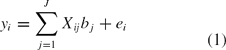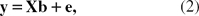The Handbook of Marketing Research: Uses, Misuses, and Future Advances comprehensively explores the approaches for delivering market insights for fact-based decision making in a market-oriented firm. Divided into four parts, the Handbook addresses (1) the different nuances of delivering insights; (2) quantitative, qualitative, and online data gathering techniques; (3) basic and advanced data analysis methods; and (4) the substantial marketing issues that clients are interested in resolving through marketing research.

# Latent Structure Regression

### Latent Structure Regression

Latent structure regression

Ordinary least squares (OLS), or multiple regression as it is also designated, is the most popular method in applied marketing research to summarize the relationship between a predesignated set of independent variables and a single dependent variable. To set the scene and establish notation for the subsequent exposition, we start to briefly summarize this well-known method. Let

• i = 1, …, I consumers,
• j = 1, …, J independent variables,
• Yi = the value of the dependent variable for consumer i,
• Xij = the value of the jth independent variable for consumer i,
• bj = the value of the jth OLS regression coefficient,
• ei = error for consumer i.

Then, the standard linear multiple regression model can be expressed asor, in matrix form,where y = ((yi)), X ...

•••